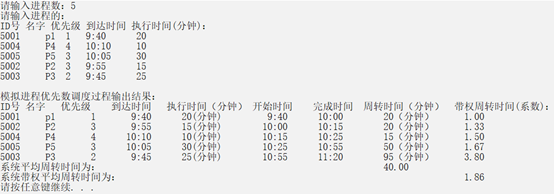#include
#include
typedef struct data
{
int hour;
int min;
}time;
typedef struct node
{
int id;//编号
char name;//名字
int good;//优先级
time arrive;//到达时间
int zx;//执行时间
time start;//开始时间
time finish;//完成时间
int zz;//周转时间
float zzxs;//带权周转系数
struct node* next;

/**********构建链表***************/
{
int num,i;
printf("请输入进程数:");
scanf("%d",&num);
printf("\n请输入进程的:");
printf("\nID号\t名字\t优先级\t到达时间\t执行时间(分钟):\n");
for(i=0;i {
else
r->next=s;
r=s;
scanf("%d\t%s\t%d\t%d:%d\t%d\n",&s->id,s->name,&s->good,&s->arrive.hour,&s->arrive.min,&s->zx);
}
if(s) s->next=NULL;
}
/*********优先数调度算法***********/
{
if(r->next==NULL || r->next->next==NULL) return ;
while(r->next)
{
q=r;
r=r->next;
r->start.hour=r->arrive.hour;
r->start.min=r->arrive.min;
}
else{
while(q->good < r->good)
{
q->start.hour=r->finish.hour;
q->start.min=r->finish.min;
}
}
r->finish.min=r->start.min+r->zx;//完成时间=开始时间+执行时间
if(r->finish.min>=60){
r->finish.hour=r->start.hour+r->finish.min/60;
r->finish.min=r->finish.min%60;
}
else
r->finish.hour=r->start.hour;
r->zz=(r->finish.hour-r->arrive.hour)*60 + r->finish.min-r->arrive.min;//周转时间
r->zzxs=(float)r->zz/r->zx;//带权周转系数
}
}
/********打印函数*********/
{
q=p->next;
printf("模拟优先数调度过程输出结果:\n");
printf("ID号\t名字\t优先数\t到达时间\t执行时间(分钟)\t开始时间\t完成时间\t周转时间(分钟)带权周转时间(系数)\t");
while(q)
{
printf("%d\t%s\t%d\t%d:%d\t%d\t%d:%d\t%d:%d\t%d(分钟)\t%f\n",p->id,p->name,p->arrive.hour,p->arrive.min,p->zx,p->start.hour,p->start.min,p->finish.hour,p->finish.min,p->zz,p->zzxs);
p=p->next;
}
}
/********主函数*********/
int main()
{